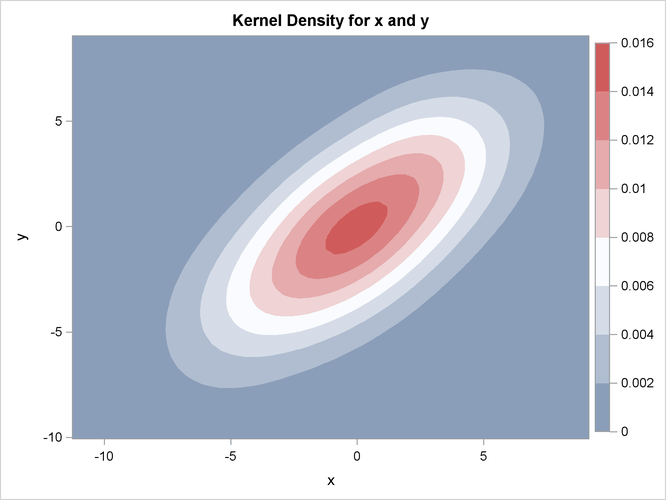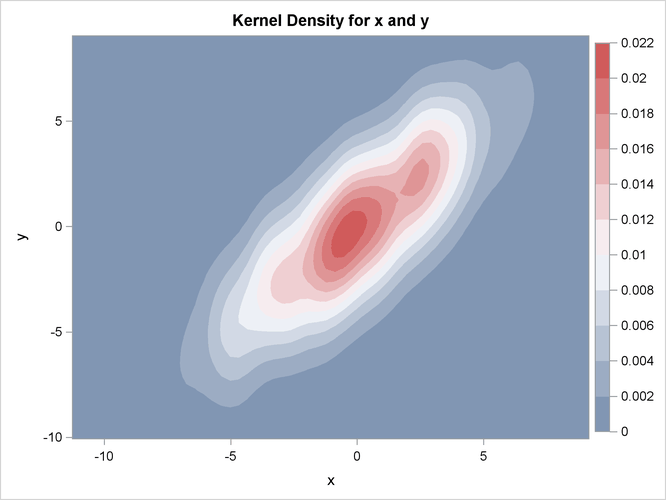# The KDE Procedure

### Example 48.3 Changing the Bandwidth (Bivariate)

Recall the analysis from the section Getting Started: KDE Procedure. Suppose you would like a slightly smoother estimate. You could then rerun the analysis with a larger bandwidth:

```ods graphics on;
proc kde data=bivnormal;
bivar x y / bwm=2;
run;
```

The BWM= option requests bandwidth multipliers of 2 for both `x` and `y`. With ODS Graphics enabled, the BIVAR statement produces a contour plot, as shown in Output 48.3.1.

Output 48.3.1: Contour Plot of Estimated Density with Additional Smoothing#### Multiple Bandwidths

You can also specify multiple bandwidths with only one run of the KDE procedure. Notice that by specifying pairs of variables inside parentheses, a kernel density estimate is computed for each pair. In the following statements the first kernel density is computed with the default bandwidth, but the second kernel density specifies a bandwidth multiplier of 0.5 for the variable `x` and a multiplier of 2 for the variable `y`:

```proc kde data=bivnormal;
bivar (x y) (x (bwm=0.5) y (bwm=2));
run;
ods graphics off;
```

The contour plot of the second kernel density estimate is shown in Output 48.3.2.

Output 48.3.2: Contour Plot of Estimated Density with Different Smoothing for `x` and `y`